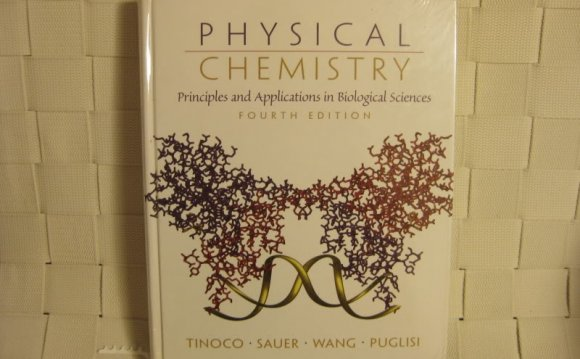# Applied Mathematics for Physical Chemistry

May 7, 20221. Coordinate Systems.

Cartesian Coordinates. Plane Polar Coordinates. Spherical Polar Coordinates. Complex Numbers.

2. Functions and Graphs.

Functions. Graphical Representation of Functions. Roots to Polynomial Equations.

3. Logarithms.

General Properties of Logarithms. Common Logarithms. Natural Logarithms.

4. Differential Calculus.

Functions of Single Variables. Functions of Several Variables-Partial Derivatives. The Total Differential. Derivative as a Ratio of Infinitesimally Small Changes. Geometric Properties of Derivatives. Constrained Maxima and Minima.

5. Integral Calculus.

Integral as an Antiderivative. General Methods of Integration. Special Methods of Integration. The Integral as a Summation of Infinitesimally Small Elements. Line Integrals. Double and Triple Integrals.

6. Infinite Series.

Tests for Convergence and Divergence. Power Series Revisited. Maclaurin and Taylor Series. Fourier Series and Fourier Transforms.

7. Differential Equations.

Linear Combinations. First-Order Differential Equations. Second-Order Differential Equations. with Constant Coefficients. General Series Methods of Solution. Special Polynomial Solutions to Differential Equations. Exact and Inexact Differentials. Integrating Factors. Partial Differential Equations.

8. Scalars and Vectors.

Addition of Vectors. Multiplication of Vectors. Applications.

9. Matrices and Determinants.

Square Matrices and Determinants. Matrix Algebra.

10. Operators.

Vector Operators. Eigenvalue Equations Revisited. Hermitian Operators. Rotational Operators. Transformation of ∇2 to Plane Polar Coordinates.

11. Numerical Methods and the Use of the Computer.

Graphical Presentation. Numerical Integration. Roots to Equations. Fourier Transforms Revisited-Macros.

12. Mathematical Methods in the Laboratory.

Probability. Experimental Errors. Propagation of Errors. Preparation of Graphs. Linear Regression. Tangents and Areas.

Appendix I. Table of Physical Constants.

Appendix II. Table of Integrals.

Source: www.barnesandnoble.com